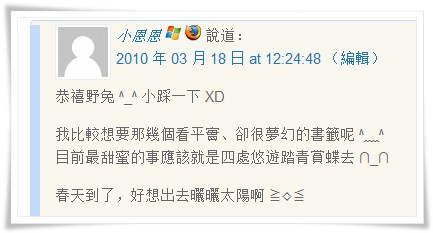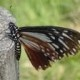2010/4/11

## 第四週迴響大樂透

55+31+03+15+50+28+57+28+53+48+55+14+57+
39+21+17+39+12+49+30+6+27+1+31+14+28+43+32 = 883

883 / 28 = 93 …餘 152010/4/3

## 第三週迴響大樂透

55+31+03+15+50+28+57+28+53+48+55+14+57+
39+21+17+39+12+49+30+6+27+1+31+14+28 = 808

808*3 / 26 = 93 …餘 62010/3/27

## 第二週迴響大樂透

55+31+03+15+50+28+57+28+53+48+55+14+57+
39+21+17+39+12+49+30+6+27+1+31+14+28 = 808

808 / 26 = 31 …餘 1 ，恭喜頭香迴響啦！！2010/3/20

## 第一週迴響大樂透

55+31+03+15+50+28+57+28+53+48+55+14+57+39+21+17+39+12 = 622

622 / 18 = 34 …餘 10 ，就是第十篇迴響啦！1. 天啊….居然雀屏中選 ☆_☆

看到野兔傳來的訊息…..還以為是詐騙集團(太會想像了XD)~~
不敢相信自己的眼睛,再三確認…哈哈,真的中了耶≧◇≦
比中樂透還要開心 ^ ^ 因為本來就很想要那個美美的書籤 ^﹏^

再次感謝野兔精心設計這份讓人幸福滿滿的小小樂透 ∩_∩
嗯!!真的好高興哦 ^▽^小恩恩1. @小恩恩：
恭喜你呀！野兔2. 歐耶~歐耶!!
或許是因為兔妹的布丁有加持的效果!!
雖然沒辦法吃到~但是光看到照片就有加持效果無誤XD

——————-
至於甜蜜事的梗!!
http://terry0912.pixnet.net/blog/post/30656648
這篇的最後

其實很多小細節都吧
不過寫個最出得起源好了

其實真的是因為躲雨
天空的閃電一閃
我看他覺得很可愛阿~無誤!!泰瑞先生1. @泰瑞先生：
恭喜泰瑞呀，呵！
最後的十萬踩踩樂也要用力踩下去啦！野兔3. 👿妞1. @妞：
妞，乖啊！野兔4. 😮 耶, 中獎囉 🙂

看來, 小兔也快來囉 😛dllee1. @dllee：
哈哈，恭喜呀！
不過小兔還是晚一點再來吧，呵野兔5. 謝謝野兔，好美的書籤耶
太感動了QQ芸兒1. @芸兒：
恭喜得獎呀！野兔6. 書籤已收到囉 😳

感謝野兔 😀dllee1. @dllee：
希望dllee會喜歡 ^^野兔